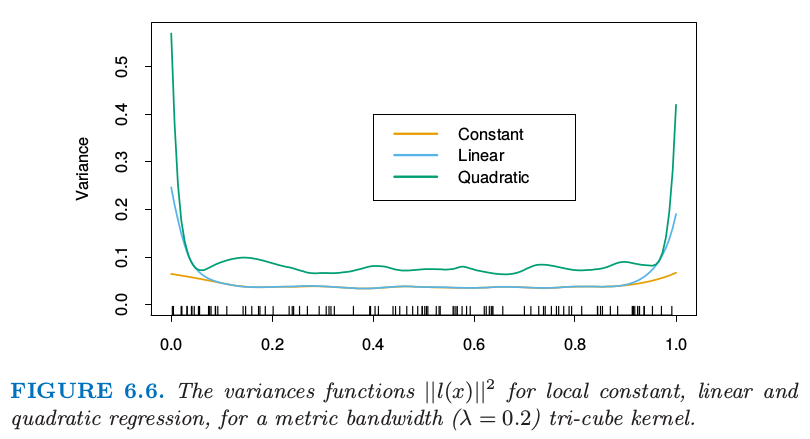6.1 一维核光滑器¶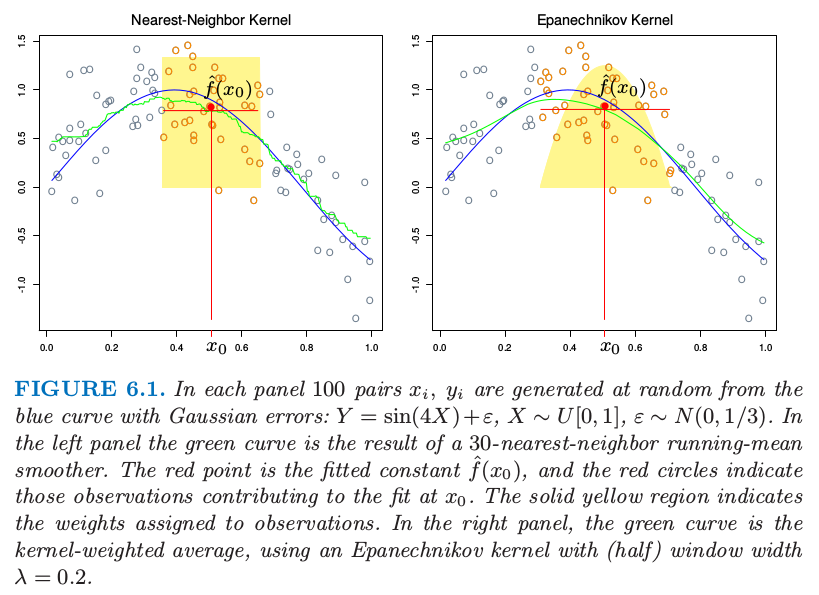• 需要确定光滑参数 $\lambda$，它决定了局部邻域的宽度．大 $\lambda$ 表明低方差（在更多的观测上平均）但高偏差（实质上假设在窗口中真实函数为常值的）．

• 度量窗口宽度（常值 $h_\lambda(x)$）趋向于保持估计的偏差为常值，但是方差与局部密度成反比．最近邻窗口宽度表现出相反地行为；方差为常值并且绝对偏差随局部密度反比例变化．

• 当在 $x_i$ 处出现 结 (tie)，最近邻出现了问题．大部分的光滑技巧，可以简单地通过在打结的 $X$ 值处对 $y_i$ 平均来减少数据集，并且对 $x_i$ 处新的唯一观测赋予额外的权重 $w_i$（这个乘以核权重）．

weiya 注：tied data

• 有个更一般的问题需要处理：观测的权重 $w_i$．实际操作中我们在计算加权平均时简单地将它们乘上核权重．在最近邻中，保持邻域的总权重 $k$（与 $\sum w_i$ 相对）是自然的．在 超出 (overflow) 的情形中（邻域中最后一个需要的观测的权重 $w_j$ 会导致权重中和超出 $k$）,于是可以采用其中的一部分（也就相当于去掉超出的量）．

• 产生边界问题．度量邻域趋向于在边界处包含更少的点，而最近邻趋向于变得更宽．

• Epanechnikov 核有紧的支撑集（当与最近邻窗口宽度同时使用会需要）．另外一个受欢迎的紧核 (compact kernel) 基于三次立方函数 这在上面是平的（与最近邻盒子类似）并且在支撑集的边界是可导的．高斯密度函数 $D(t)=\phi(t)$ 是受欢迎的非紧核，其中标准误差扮演了窗口大小的角色．图 6.2 比较了这三个．

weiya 注：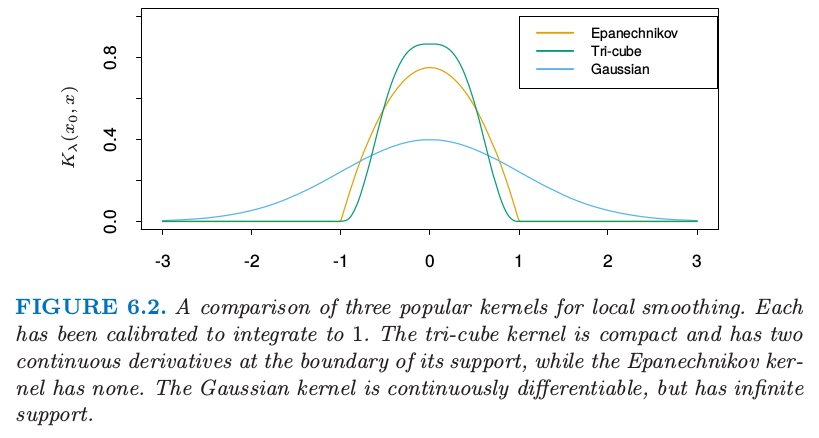局部线性回归¶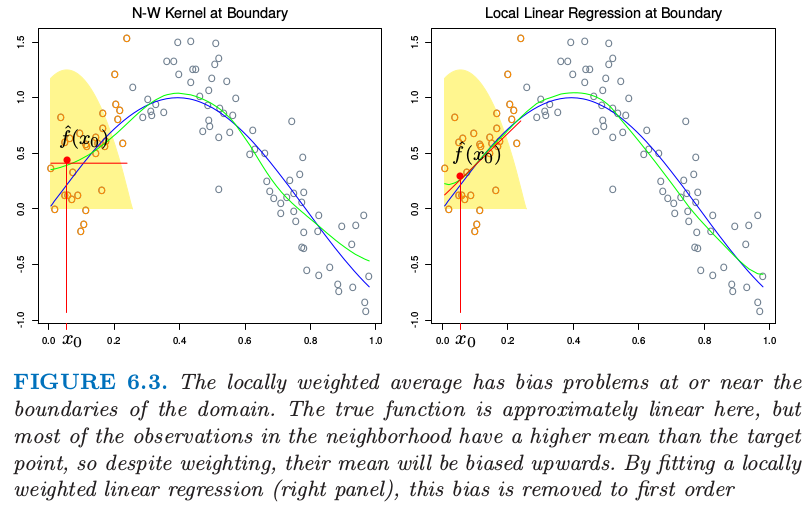weiya 注：不对称性

weiya 注

weiya 注：automatic kernel carpentry

weiya 注：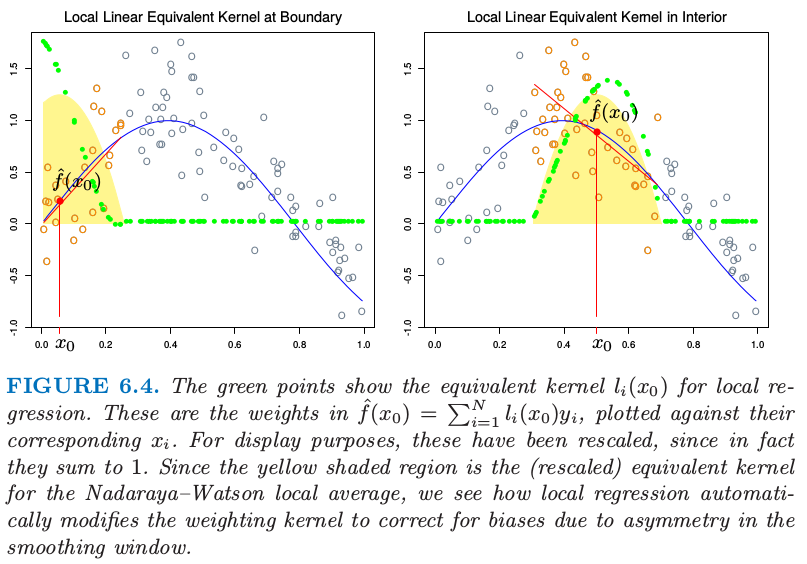局部多项式回归¶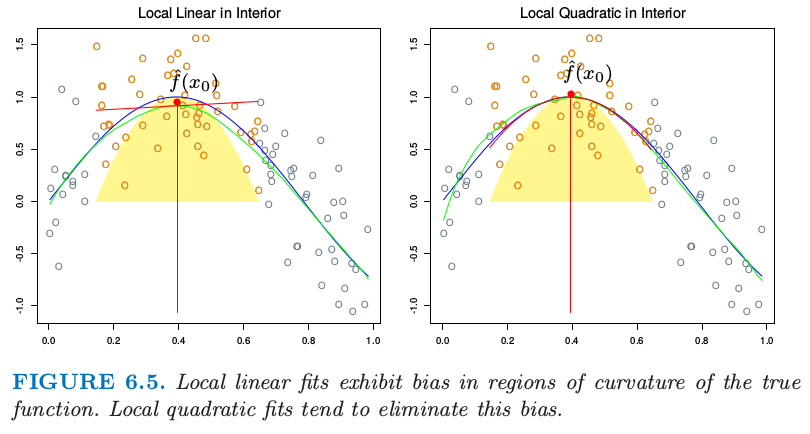weiya 注：Ex. 6.3

• 局部线性拟合在边界处以适合的方差代价对偏差有显著的帮助．但局部二次拟合在边界处对偏差的贡献很少，而且大幅增加了方差．
• 局部二次拟合在定义域内部对于降低由于曲率造成的偏差趋向于是最有帮助的．
• 渐近分析表明奇数阶的局部多项式表现得比偶数阶要好．大部分是因为渐近情况下，MSE 由边界影响主导．